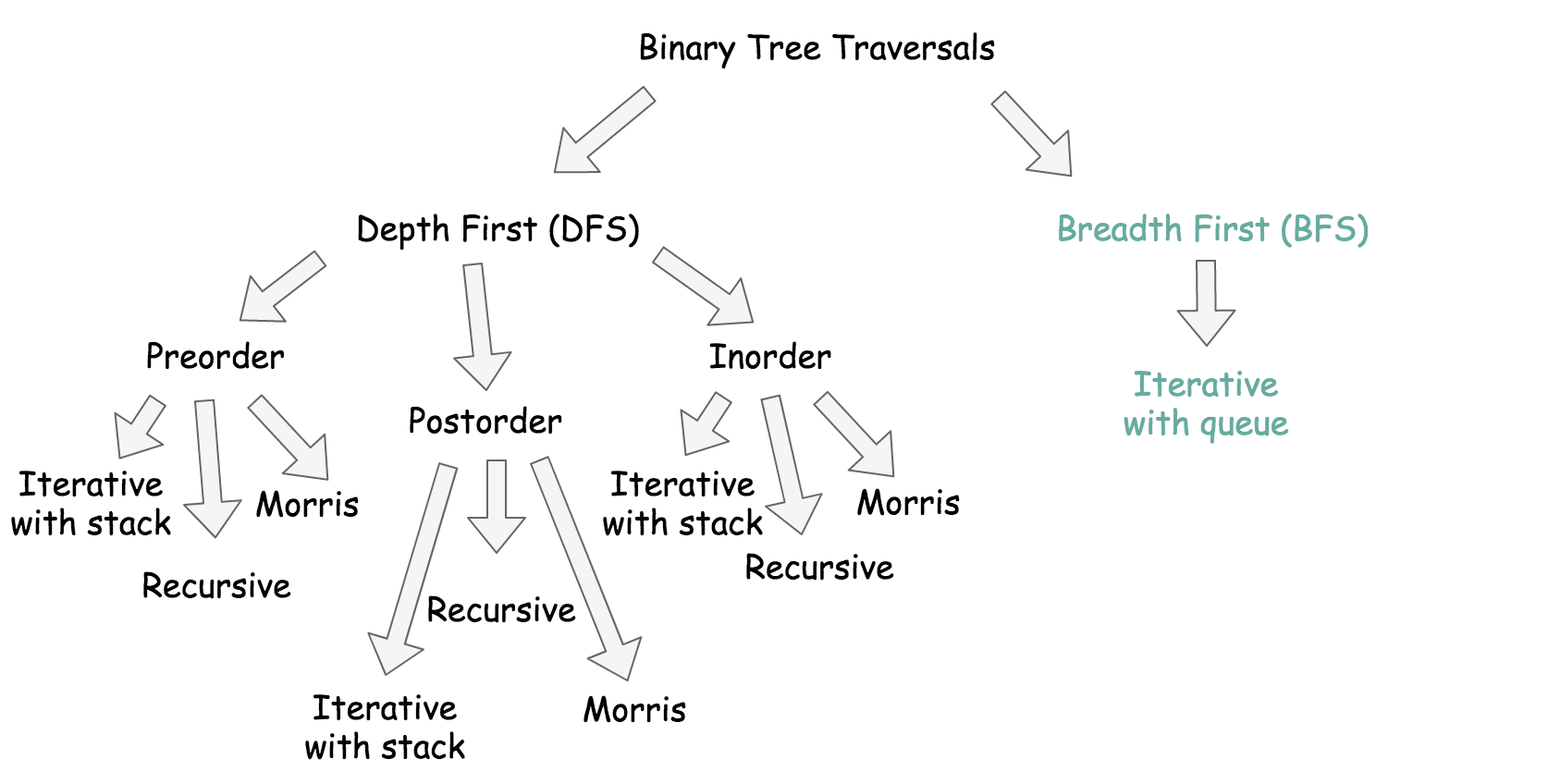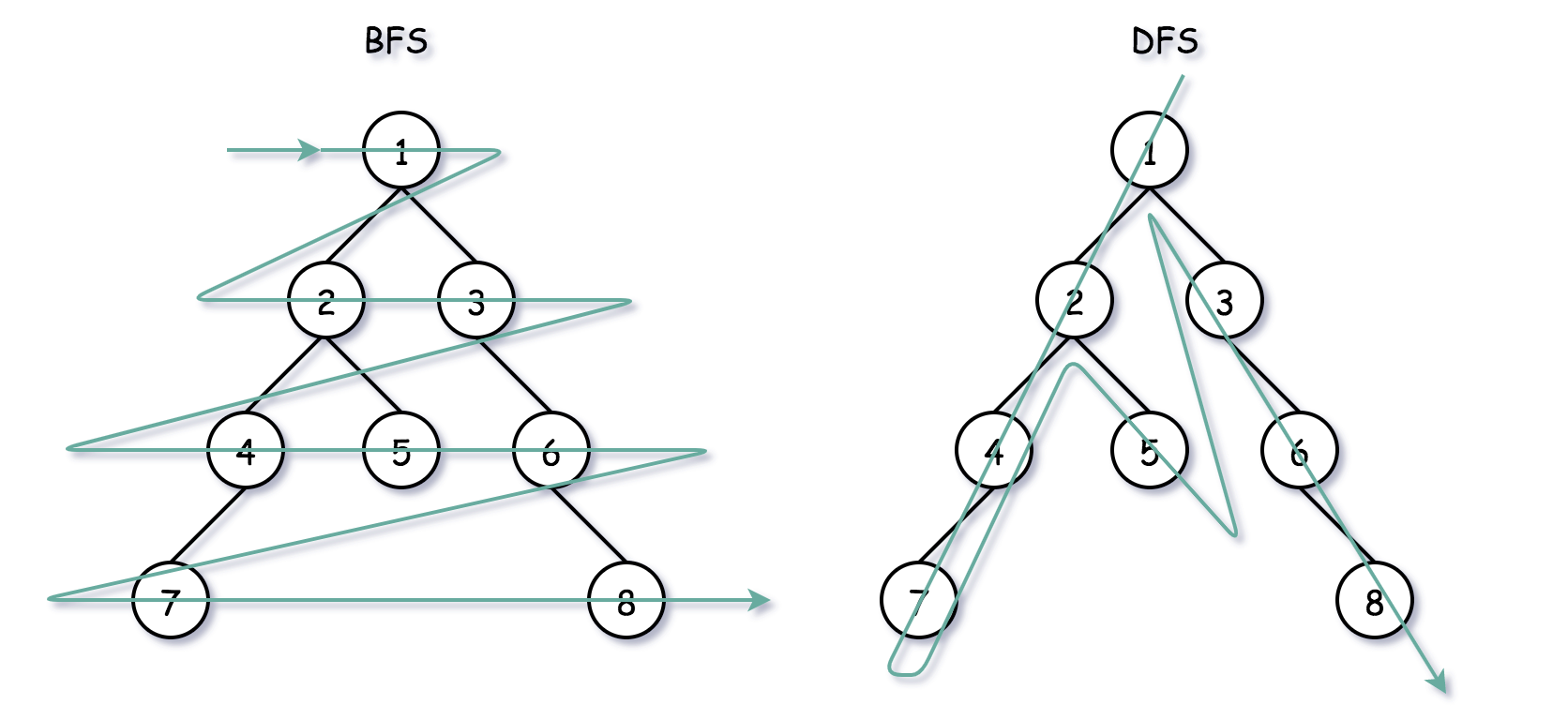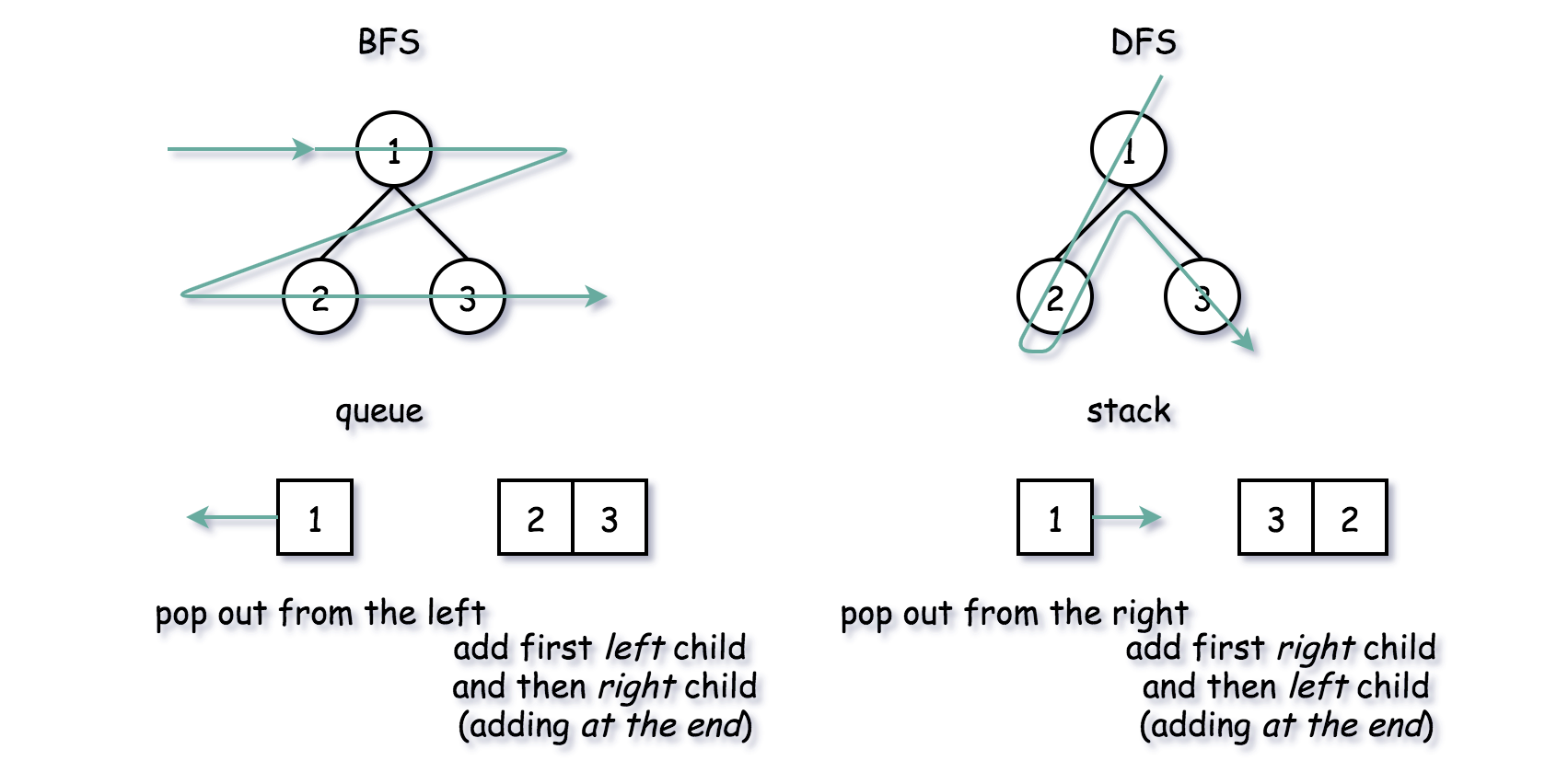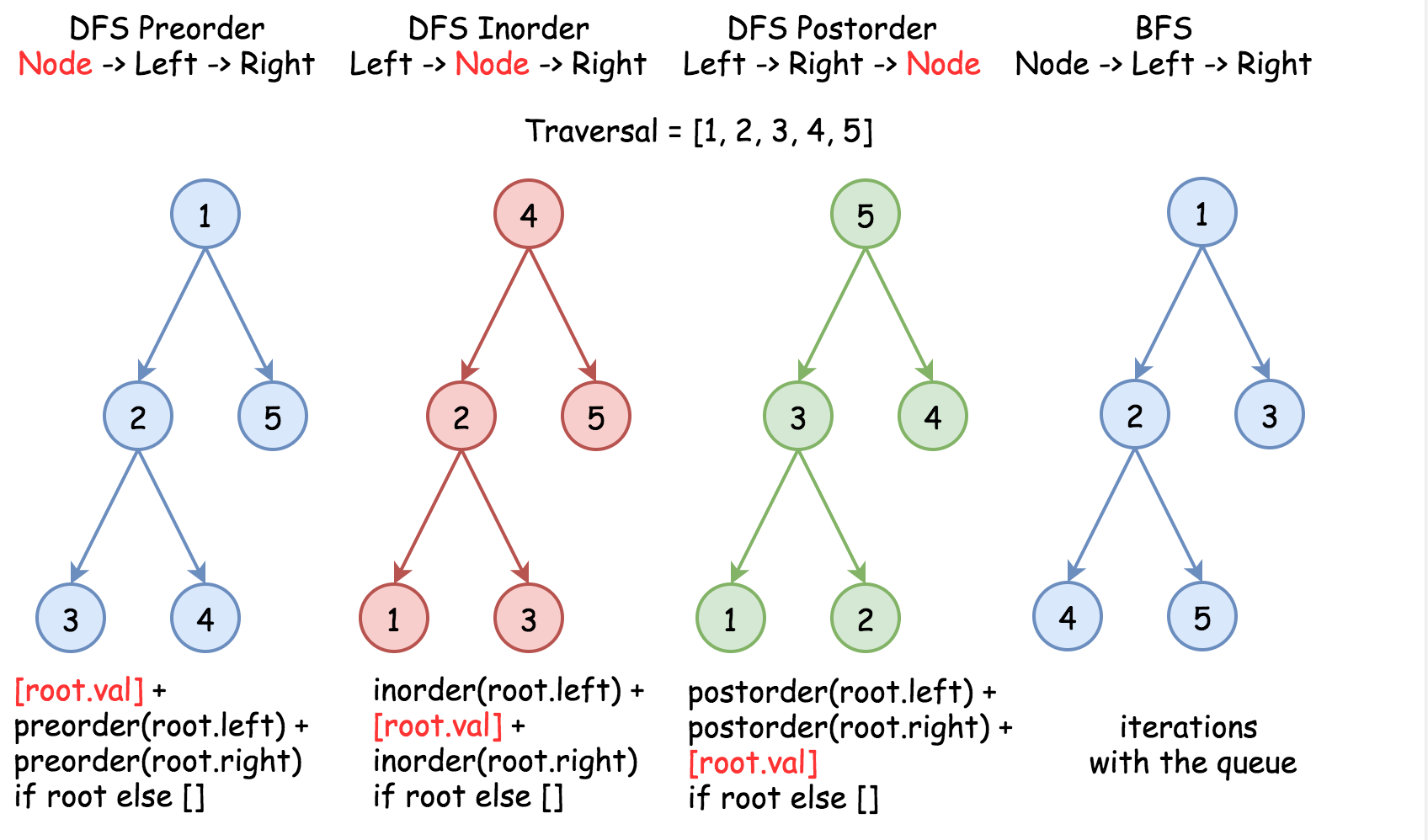## DFS vs. BFS

• There are two ways to traverse the tree: Depth First Search (DFS) and Breadth First Search (BFS). Here is a small summary:• Key takeaways:
• There are two general strategies to traverse a tree:
• DFS:
• In this strategy, we adopt the depth as the priority, so that one would start from a root and reach all the way down to certain leaf, and then back to root to reach another branch.
• The DFS strategy can further be distinguished as preorder, inorder, and postorder depending on the relative order among the root node, left node and right node.
• BFS:
• We scan through the tree level by level, following the order of height, from top to bottom. The nodes on higher level would be visited before the ones with lower levels.
• In summary, BFS traverses level by level, and DFS goes deeper into the leaves.• Which approach to choose, BFS or DFS?

• If the problem is to return a list of last elements from all levels, the more natural way to implement it is using BFS. On the other hand, if the problem is to calculate the number of permutations/combinations, the more natural way to implement it is using DFS.
• Time complexity is the same $$\mathcal{O}(N)$$ both for DFS and BFS since one has to visit all nodes.
• Space complexity is $$\mathcal{O}(H)$$ for DFS and $$\mathcal{O}(D)$$ for BFS, where $$H$$ is a tree height, and $$D$$ is a tree diameter. They both result in $$\mathcal{O}(N)$$ space in the worst-case scenarios: skewed tree for DFS and **complete tree for BFS.
• Standard BFS implementation:
• Push the root into the queue.
• Pop-out a node from the left.
• Push the left child into the queue, and then push the right child.
• Standard DFS implementation:
• Push the root into the stack.
• Pop-out a node from the right (top of the stack).
• Push the right child into the stack, and then push the left child.• In the following figure, the nodes are numerated in the order you visit them, please follow 1-2-3-4-5 to compare different strategies.